# 题目 127

A transformation sequence from word beginWord to word endWord using a dictionary wordList is a sequence of words beginWord -> s1 -> s2 -> … -> sk such that:

Every adjacent pair of words differs by a single letter.
Every si for 1 <= i <= k is in wordList. Note that beginWord does not need to be in wordList.
sk == endWord


Given two words, beginWord and endWord, and a dictionary wordList, return the number of words in the shortest transformation sequence from beginWord to endWord, or 0 if no such sequence exists.

Example 1:

Input: beginWord = "hit", endWord = "cog", wordList = ["hot","dot","dog","lot","log","cog"]
Output: 5
Explanation: One shortest transformation sequence is "hit" -> "hot" -> "dot" -> "dog" -> cog", which is 5 words long.


Example 2:

Input: beginWord = "hit", endWord = "cog", wordList = ["hot","dot","dog","lot","log"]
Output: 0
Explanation: The endWord "cog" is not in wordList, therefore there is no valid transformation sequence.


## Constraints:

1 <= beginWord.length <= 10
endWord.length == beginWord.length
1 <= wordList.length <= 5000
wordList[i].length == beginWord.length
beginWord, endWord, and wordList[i] consist of lowercase English letters.
beginWord != endWord
All the words in wordList are unique.


PS：吐槽一下 127 这个题目，应该是 126 的基础，而不是反过来。

# 思路详解

A very highly detailed EXPLANATION

start = be
end = ko
words = ["ce", "mo", "ko", "me", "co"]ps: 这里还是保留原文，同时使用谷歌翻译比较好，避免混乱。

So, we intialize our queue that have starting word inside of it i.e. "be", then our changes variable is going to start at 1, this is because at minimum we going to have starting word in our minimum. And finally we have a set which will keep track's of node that have been visited, in this case we just keeping track of string that we have already added inside our queue.


queue = ["be"  ]    //存放每一个字符串
changes = 1     // 最后的结果
set = ["be"  ]  // 已经访问的节点

So, to start of our bfs, we take "be" off from our queue & we can only change one character at a time.

So, first we gonna check by changing the character b, if we can form another word inside our word list.

So, we try ae, which is not in our word list. Then, be is already in our set, so we can't use that. Now we try ce and we have that word inside our word list. With that means add ce in our queue.

Then we check de, fe and so on............ until we get to me which is inside our word list, so we add me inside our word list as well.

So, all that way we check all the way down to ze and there is no other words that we add by changing b to another character.


 所以，我们尝试 ae，它不在我们的单词列表中。 然后，be 已经在我们的集合中，所以我们不能使用它。 现在我们尝试 ce 并且我们的单词列表中有那个单词。 这意味着在我们的队列中添加 ce。

然后我们检查 de、fe 等等......直到我们找到 me ，它在我们的单词列表中，所以我们也将 me 添加到我们的单词列表中。

因此，我们一直检查到 ze ，并且没有通过将 b 更改为另一个字符来添加其他单词。


ps: 这里的比较其实有一个技巧。那就是，因为 wordList 中数量可能会比较多，所以 wordlist 中循环对比，反而是比较麻烦的办法。直接 a-z 对每一位进行变化，然后判断结果是否存在，这种性能可能比较好的。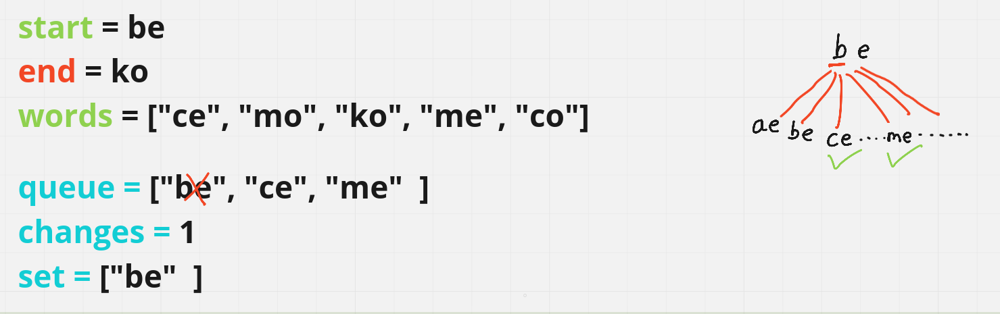So, now we need to check first index. So, by changing the character e from be to something else if we form an another word.

So, we gonna check ba, bb all the way down to bz. However none of the word inside our word list.

Adittionaly, thw word ce & me are going to get added inside our set. To show, that we have already visited those words.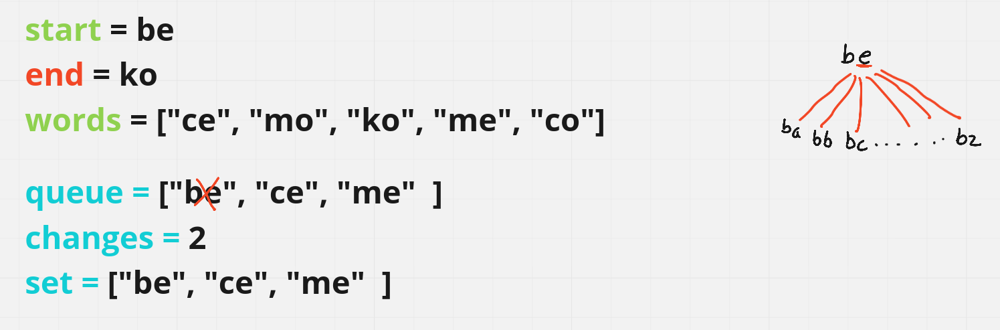PS: 就是遍历每一个字符，然后对每一位 a-z 变换。

And then we gonna perform same logic as before. We poll from our queue we take ce off from our queue. And we check if by changing any character we can form another word.

So, we gonna see if we change first character c to another character to form a word. So, we gonna try ae , be, ce which is already in our set doesn't count de, ee all the ay down to ze and none of the word included inside our word list.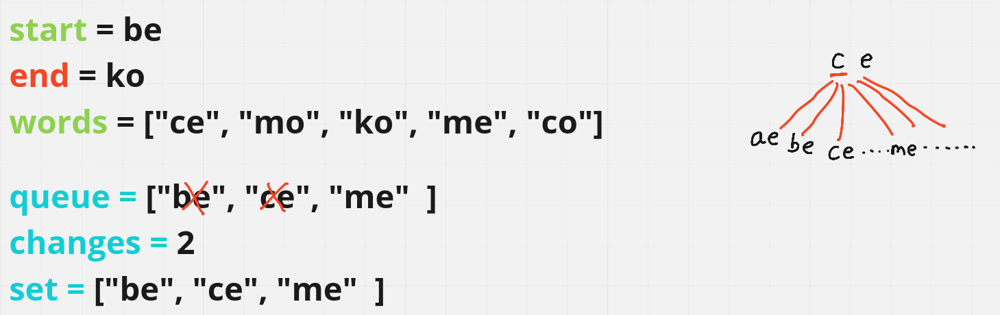Now we gonna see if we change e from ce to another character.

So, we gonna try ca, cb and so on..... eventually we get to the word co which is in our word list. So what that means we gonna add co to our queue & our set.## java 实现

public int ladderLength(String beginWord, String endWord, List<String> wordList) {
//fast-return
Set<String> wordSet = new HashSet<>(wordList);
if(!wordSet.contains(endWord)) {
return 0;
}

// 存放 BFS 元素,初始为开始词

// 已经访问过得元素
Set<String> visited = new HashSet<>();

// 变化的结果
int result = 1;

System.out.println("queue: " + queue + ";   visited: " + visited + "; result: " + result);

// 遍历整个队列
while(!queue.isEmpty()){
int size = queue.size();
for(int i = 0; i < size; i++){
// 获取队列中的第一个元素
String word = queue.poll();
// 如果和终止词相同，则迭代结束，直接返回结果。
if(word.equals(endWord)) {
return result;
}

for(int j = 0; j < word.length(); j++){
for(int k = 'a'; k <= 'z'; k++){
// 穷举 a-z 变化 word 单词的每一位，
char[] arr = word.toCharArray();
arr[j] = (char) k;

String str = new String(arr);

// 如果单词中有这个词
// 而且已经没有处理过，则加入进来。
if(wordSet.contains(str)
&& !visited.contains(str)){
// 更新 queue 中元素
// 更新访问过的元素

System.out.println("queue: " + queue + ";   visited: " + visited + "; result: " + result);
}
}
}
}

++result;
}

// 无匹配
return 0;
}


queue: [be];       visited: [be]; result: 1
queue: [ce];       visited: [ce, be]; result: 1
queue: [ce, me];   visited: [ce, be, me]; result: 1
queue: [me, co];   visited: [ce, be, me, co]; result: 2
queue: [co, mo];   visited: [ce, mo, be, me, co]; result: 2
queue: [mo, ko];   visited: [ce, mo, be, ko, me, co]; result: 3


## V2 性能优化

    public int ladderLength(String beginWord, String endWord, List<String> wordList) {
Set<String> beginSet = new HashSet<>();
Set<String> endSet = new HashSet<>();
Set<String> wordSet = new HashSet<>(wordList);
if(!wordSet.contains(endWord)) {
return 0;
}

return search(beginSet, endSet, wordSet, 1);
}

private int search(Set<String> beginSet, Set<String> endSet, Set<String> wordSet, int result){
if(beginSet.isEmpty() || endSet.isEmpty()) {
return 0;
}

result++;

// 从字典中，移除了开始的集合？
wordSet.removeAll(beginSet);

Set<String> nextSet = new HashSet<>();

// 遍历开始的 set
for(String str : beginSet){

// 这里核心算法应该是类似的。
char[] chs = str.toCharArray();
for(int i = 0; i < chs.length; i++){
char c = chs[i];
for(char j = 'a'; j <= 'z'; j++){
chs[i] = j;

// a-z 的变化开始词的每一个字符，如果字典中不存在，则跳过。
// 如果和终止词相同，则结束。

String tmp = new String(chs);
if(!wordSet.contains(tmp)) {
continue;
}
if(endSet.contains(tmp)) {
return result;
}
}
chs[i] = c;
}
}

// 这里是优化的原因吗?
// 其实最初的 endSet 中只有一个元素，那就是 endword 终止词
// 这里会进行对比，入参时交换了  endSet 和 nextSet 的顺序？？
// serch 中，最大的计算量在于对 benginSet 的 a-z 穷举变化，所以数量越小越好。

return nextSet.size() > endSet.size() ? search(endSet, nextSet, wordSet, result) : search(nextSet, endSet, wordSet, result);
}


PS: 这个为什么会快了这么多呢？

# 题目 126

A transformation sequence from word beginWord to word endWord using a dictionary wordList is a sequence of words beginWord -> s1 -> s2 -> … -> sk such that:

Every adjacent pair of words differs by a single letter.

Every si for 1 <= i <= k is in wordList. Note that beginWord does not need to be in wordList.

sk == endWord

Given two words, beginWord and endWord, and a dictionary wordList, return all the shortest transformation sequences from beginWord to endWord, or an empty list if no such sequence exists.

Each sequence should be returned as a list of the words [beginWord, s1, s2, …, sk].

## Ex

Example 1:

Input: beginWord = "hit", endWord = "cog", wordList = ["hot","dot","dog","lot","log","cog"]
Output: [["hit","hot","dot","dog","cog"],["hit","hot","lot","log","cog"]]
Explanation: There are 2 shortest transformation sequences:
"hit" -> "hot" -> "dot" -> "dog" -> "cog"
"hit" -> "hot" -> "lot" -> "log" -> "cog"


Example 2:

Input: beginWord = "hit", endWord = "cog", wordList = ["hot","dot","dog","lot","log"]
Output: []
Explanation: The endWord "cog" is not in wordList, therefore there is no valid transformation sequence.


## Constraints:

• 1 <= beginWord.length <= 5

• endWord.length == beginWord.length

• 1 <= wordList.length <= 500

• wordList[i].length == beginWord.length

• beginWord, endWord, and wordList[i] consist of lowercase English letters.

• beginWord != endWord

• All the words in wordList are unique.

• The sum of all shortest transformation sequences does not exceed 10^5.

# 第一感觉

【思路】

（1）判断 wordlist 是否包含 endword，fast-return

（2）从 endword 开始推理，依次获得每一个 word 获取所有编辑距离为1的单词。

Set: 为了提升判断 char 变化之后，wordlist 中是否存在，可以把 wordlist 首先转换为 set。查找复杂度变为 O(1)。

（3）如何回溯呢？

3.1 change1HashMap.get(endWord) 可以得到所有差距为1的映射 list，不存在则终止

3.2 遍历 3.2 中的 list，不断重复这个过程，这里就需要用到回溯。

3.3 直到最后没有映射 list，或者结果就是 starword。终止。

# 算法实现

## V1 版本

/**
* 最小路径大小
*/
private int minPathSize = Integer.MAX_VALUE;

public List<List<String>> findLadders(String beginWord, String endWord, List<String> wordList) {
List<List<String>> resultList = new ArrayList<>();
List<String> tempList = new ArrayList<>();

// 对数据提前处理？
Map<String, List<String>> changeWordMap = buildWordChangeMap(beginWord, wordList);

this.backtrack(resultList, beginWord, endWord, changeWordMap, tempList, 0);

// 最短路径，而不是所有路径？
return filterList(resultList);
}

public List<List<String>> filterList(List<List<String>>  allList) {
if(allList.size() <= 0) {
return allList;
}

// 过滤
List<List<String>> resultList = new ArrayList<>();
for(List<String> paths : allList) {
if(paths.size() <= minPathSize) {
}
}

return resultList;
}

/**
* 终止条件
*
* @param resultList 结果
* @param beginWord 开始词
* @param endWord 终止词
* @param changeWordMap 变更为1的字典映射
* @param tempList 存放临时的路径
*/
private void backtrack(List<List<String>> resultList,
String beginWord,
String endWord,
Map<String, List<String>> changeWordMap,
List<String> tempList,
int index) {
List<String> beginWordChangeOneList = changeWordMap.get(beginWord);
if(beginWordChangeOneList == null
|| tempList.size() > minPathSize) {
// 什么时候中断呢？

return;
} else if(beginWord.equals(endWord)) {
// 更新大小
if(tempList.size() < minPathSize) {
minPathSize = tempList.size();
}
// 过滤掉不是最小的

// 但是这样，还是可能会导致添加的元素长度过长
// 比如第一次长度为10， 后续越来越少，只有最小的其实才符合。
} else {
// 更新开始信息
// 在所有的剩余集合中，选择信息
for(int i = 0; i < beginWordChangeOneList.size(); i++) {
String newBeginWord = beginWordChangeOneList.get(i);
// 这里有一个问题，其实单词不应该被重复使用，否则会死循环。
if(tempList.contains(newBeginWord)) {
continue;
}

// 什么时候回溯？
backtrack(resultList, newBeginWord, endWord, changeWordMap, tempList, index+1);
// backtrack
tempList.remove(tempList.size()-1);
}
}
}

/**
* 构建1个变换量的词库映射
*
* wordlist 不超过 500 个。
* 如何找到变化为1的单词呢？
*
* 其实也就是2个单词不同的字母只有1个。
*
*
*
* 【发现测试用例7，并不需要保证次序】
* PS: 这里这种处理存在一个问题，那就是必须在后边的才行。
* 从而保证一个顺序：beginWord -> s1 -> s2 -> ... -> sk
*
* @param beginWord 开始词
* @param wordList 单词列表
* @return 结果
*/
private Map<String, List<String>> buildWordChangeMap(String beginWord, List<String> wordList) {
Map<String, List<String>> resultMap = new HashMap<>();

// 开始词
resultMap.put(beginWord, getMappingList(beginWord,  wordList));

// 2 次迭代
for(int i = 0; i < wordList.size(); i++) {
String s = wordList.get(i);
List<String> tempList = getMappingList(s, wordList);
resultMap.put(s, tempList);
}

return resultMap;
}

private List<String> getMappingList(String word, List<String> wordList) {
List<String> tempList = new ArrayList<>();

for(int i = 0; i < wordList.size(); i++) {
String t = wordList.get(i);

// 差距为1的单词
if(isDifferOne(word, t)) {
}
}

return tempList;
}

/**
* 两个字符的差距是否为 1
* @param s 原始
* @param t 目标
* @return 结果
*/
private boolean isDifferOne(String s, String t) {
int differCount = 0;
for(int i = 0; i < s.length(); i++) {
char sc = s.charAt(i);
char tc = t.charAt(i);

if(sc != tc) {
differCount++;
}
}

return differCount == 1;
}


## V2 如何优化性能？

beginWord ="qa"
endWord = "sq"
wordList =
["si","go","se","cm","so","ph","mt","db","mb","sb","kr","ln","tm","le","av","sm","ar","ci","ca","br","ti","ba","to","ra","fa","yo","ow","sn","ya","cr","po","fe","ho","ma","re","or","rn","au","ur","rh","sr","tc","lt","lo","as","fr","nb","yb","if","pb","ge","th","pm","rb","sh","co","ga","li","ha","hz","no","bi","di","hi","qa","pi","os","uh","wm","an","me","mo","na","la","st","er","sc","ne","mn","mi","am","ex","pt","io","be","fm","ta","tb","ni","mr","pa","he","lr","sq","ye"]


import java.util.*;

/**
* 最小路径大小
*/
private int minPathSize = 0;

public List<List<String>> findLadders(String beginWord, String endWord, List<String> wordList) {
// dfs 首先获取最小路径

List<List<String>> resultList = new ArrayList<>();
// 快速失败
if(minPathSize <= 0) {
return resultList;
}

List<String> tempList = new ArrayList<>();

// 对数据提前处理？
//map 构建几毫秒，耗时不多。不过应该可以优化。
Map<String, List<String>> changeWordMap = buildWordChangeMap(beginWord, wordList);

this.backtrack(resultList, beginWord, endWord, changeWordMap, tempList, 0);

// 最短路径，而不是所有路径？
return resultList;
}

/**
* 127 中的，获取最小路径
* @param beginWord 开始
* @param endWord 结束
* @param wordList 单词字典
* @return 结果
*/
private int ladderLength(String beginWord, String endWord, List<String> wordList) {
Set<String> beginSet = new HashSet<>();
Set<String> endSet = new HashSet<>();
Set<String> wordSet = new HashSet<>(wordList);
if(!wordSet.contains(endWord)) {
return 0;
}

return bfs(beginSet, endSet, wordSet, 1);
}

private int bfs(Set<String> beginSet, Set<String> endSet, Set<String> wordSet, int result){
if(beginSet.isEmpty() || endSet.isEmpty()) {
return 0;
}

result++;

// 从字典中，移除了开始的集合？
wordSet.removeAll(beginSet);

Set<String> nextSet = new HashSet<>();

// 遍历开始的 set
for(String str : beginSet){

// 这里核心算法应该是类似的。
char[] chs = str.toCharArray();
for(int i = 0; i < chs.length; i++){
char c = chs[i];
for(char j = 'a'; j <= 'z'; j++){
chs[i] = j;

// a-z 的变化开始词的每一个字符，如果字典中不存在，则跳过。
// 如果和终止词相同，则结束。

String tmp = new String(chs);
if(!wordSet.contains(tmp)) {
continue;
}
if(endSet.contains(tmp)) {
return result;
}
}
chs[i] = c;
}
}
// 这里是优化的原因吗?
// 其实最初的 endSet 中只有一个元素，那就是 endword 终止词
// 这里会进行对比，入参时交换了  endSet 和 nextSet 的顺序？？
// serch 中，最大的计算量在于对 benginSet 的 a-z 穷举变化，所以数量越小越好。

return nextSet.size() > endSet.size() ? bfs(endSet, nextSet, wordSet, result) : bfs(nextSet, endSet, wordSet, result);
}

/**
* 终止条件
*
* @param resultList 结果
* @param beginWord 开始词
* @param endWord 终止词
* @param changeWordMap 变更为1的字典映射
* @param tempList 存放临时的路径
*/
private void backtrack(List<List<String>> resultList,
String beginWord,
String endWord,
Map<String, List<String>> changeWordMap,
List<String> tempList,
int index) {
List<String> beginWordChangeOneList = changeWordMap.get(beginWord);
if(beginWordChangeOneList == null
|| tempList.size() > minPathSize) {
// 什么时候中断呢？
return;
} else if(beginWord.equals(endWord)) {
// 过滤掉不是最小的
// 什么时候符合结果？

// 但是这样，还是可能会导致添加的元素长度过长
// 比如第一次长度为10， 后续越来越少，只有最小的其实才符合。

} else {
// 更新开始信息
// 在所有的剩余集合中，选择信息
for(int i = 0; i < beginWordChangeOneList.size(); i++) {
String newBeginWord = beginWordChangeOneList.get(i);
// 这里有一个问题，其实单词不应该被重复使用，否则会死循环。
if(tempList.contains(newBeginWord)) {
continue;
}

// 什么时候回溯？
backtrack(resultList, newBeginWord, endWord, changeWordMap, tempList, index+1);
// backtrack
tempList.remove(tempList.size()-1);
}
}
}

/**
* 构建1个变换量的词库映射
*
* wordlist 不超过 500 个。
* 如何找到变化为1的单词呢？
*
* 其实也就是2个单词不同的字母只有1个。
*
*
*
* 【发现测试用例7，并不需要保证次序】
* PS: 这里这种处理存在一个问题，那就是必须在后边的才行。
* 从而保证一个顺序：beginWord -> s1 -> s2 -> ... -> sk
*
* @param beginWord 开始词
* @param wordList 单词列表
* @return 结果
*/
private Map<String, List<String>> buildWordChangeMap(String beginWord, List<String> wordList) {
Map<String, List<String>> resultMap = new HashMap<>();

// 开始词
resultMap.put(beginWord, getMappingList(beginWord,  wordList));

// 2 次迭代
for(int i = 0; i < wordList.size(); i++) {
String s = wordList.get(i);
List<String> tempList = getMappingList(s, wordList);
resultMap.put(s, tempList);
}

return resultMap;
}

private List<String> getMappingList(String word, List<String> wordList) {
List<String> tempList = new ArrayList<>();

for(int i = 0; i < wordList.size(); i++) {
String t = wordList.get(i);

// 差距为1的单词
if(isDifferOne(word, t)) {
}
}

return tempList;
}

/**
* 两个字符的差距是否为 1
* @param s 原始
* @param t 目标
* @return 结果
*/
private boolean isDifferOne(String s, String t) {
int differCount = 0;
for(int i = 0; i < s.length(); i++) {
char sc = s.charAt(i);
char tc = t.charAt(i);

if(sc != tc) {
differCount++;
}
}

return differCount == 1;
}

}


beginWord =
"cet"
endWord =
"ism"
wordList =


## 性能优化 V3

BFS + DFS

he basic idea is:

1). Use BFS to find the shortest distance between start and end, tracing the distance of crossing nodes from start node to end node, and store node's next level neighbors to HashMap;

2). Use DFS to output paths with the same distance as the shortest distance from distance HashMap: compare if the distance of the next level node equals the distance of the current node + 1.


java 代码：

public class T126_WordLadderIIV_OTHER2 {

public List<List<String>> findLadders(String start, String end, List<String> wordList) {
HashSet<String> dict = new HashSet<>(wordList);
List<List<String>> res = new ArrayList<>();
// Neighbors for every node
HashMap<String, List<String>> nodeNeighbors = new HashMap<>();
// Distance of every node from the start node
HashMap<String, Integer> distance = new HashMap<>();
ArrayList<String> solution = new ArrayList<>();

bfs(start, end, dict, nodeNeighbors, distance);
dfs(start, end, nodeNeighbors, distance, solution, res);
return res;
}

/**
* BFS: Trace every node's distance from the start node (level by level).
* 1. 计算出最小路径长度
* 2. 记录临边和对应的长度
* @param start 开始词
* @param end 结束词
* @param dict 字典
* @param nodeNeighbors 临边
* @param distance 距离
*/
private void bfs(String start, String end, Set<String> dict,
HashMap<String, List<String>> nodeNeighbors,
HashMap<String, Integer> distance) {
for (String str : dict) {
nodeNeighbors.put(str, new ArrayList<>());
}
queue.offer(start);
distance.put(start, 0);

while (!queue.isEmpty()) {
int count = queue.size();
boolean foundEnd = false;
for (int i = 0; i < count; i++) {
String cur = queue.poll();
int curDistance = distance.get(cur);
// 这里构建的可达边，比直接初始化构建要少很多。
ArrayList<String> neighbors = getNeighbors(cur, dict);

for (String neighbor : neighbors) {
// Check if visited
if (!distance.containsKey(neighbor)) {
distance.put(neighbor, curDistance + 1);
// Found the shortest path
if (end.equals(neighbor))
{
foundEnd = true;
} else {
queue.offer(neighbor);
}
}
}
}

if (foundEnd) {
break;
}
}
}

// Find all next level nodes.
private ArrayList<String> getNeighbors(String node, Set<String> dict) {
ArrayList<String> res = new ArrayList<>();
char[] chs = node.toCharArray();

for (char ch = 'a'; ch <= 'z'; ch++) {
for (int i = 0; i < chs.length; i++) {
if (chs[i] == ch) {
continue;
}
char oldCh = chs[i];
chs[i] = ch;
if (dict.contains(String.valueOf(chs))) {
}
chs[i] = oldCh;
}

}
return res;
}

// DFS: output all paths with the shortest distance.
private void dfs(String cur, String end, HashMap<String, List<String>> nodeNeighbors,
HashMap<String, Integer> distance,
List<String> solution,
List<List<String>> res) {
if (end.equals(cur)) {
} else {
for (String next : nodeNeighbors.get(cur)) {
if (distance.get(next) == distance.get(cur) + 1) {
dfs(next, end, nodeNeighbors, distance, solution, res);
}
}
}
solution.remove(solution.size() - 1);
}

}


beginWord =
"aaaaa"
endWord =
"ggggg"
wordList =


## 最快的算法

fastest

class Solution {
List<List<String>> ans = new ArrayList<>();
Map<String, Integer> wordToId = new HashMap<>();
Map<Integer, String> idToWord = new HashMap<>();
Map<Integer, List<Integer>> path = new HashMap<Integer, List<Integer>>();
int[] ne, e, h;
boolean[] vis;
int len, idx, start, end;
void add(int u, int v) {
e[++len] = v;
ne[len] = h[u];
h[u] = len;
}
public List<List<String>> findLadders(String beginWord, String endWord, List<String> wordList) {
int n = wordList.size();
ne = new int[20 * n];
e = new int[20 * n];
h = new int[20 * n];
vis = new boolean[10 * n];
if (!wordList.contains(endWord)) return ans;
for (int i = 0; i < wordList.size(); i++) addEdge(wordList.get(i));
start = wordToId.get(beginWord);
end = wordToId.get(endWord);
while (!q.isEmpty()) {
int u = q.poll();
if (u == end) break;
if (vis[u]) continue;
vis[u] = true;
for (int j = h[u]; j != 0; j = ne[j]) {
int v = e[j];
if (vis[v]) continue;
if (!path.containsKey(v)) path.put(v, new ArrayList<>());
}
}
//从终点dfs搜索出路径
dfs(end, 0);
return ans;
}
void dfs(int u, int level) {
if (u == start) {
//到达起点
return;
}
List<Integer> p = path.get(u);
if (p == null) return;
for (int i = 0; i < p.size(); i++) {
int v = p.get(i);
if (level % 2 == 1) list.addFirst(idToWord.get(v));
dfs(v, level + 1);
if (level % 2 == 1) list.pollFirst();
}
}
int u = idx;
char[] arr = word.toCharArray();
wordToId.put(word, idx);
idToWord.put(idx++, word);
for (int i = 0; i < arr.length; i++) {
char t = arr[i];
arr[i] = '*';
String vstr = new String(arr);
if (!wordToId.containsKey(vstr)) {
wordToId.put(vstr, idx);
idToWord.put(idx++, vstr);
}
int v = wordToId.get(vstr);
arr[i] = t;
}
}
}


# 他山之石1

Explanation with Animation - Accepted without TLE!

## 直观图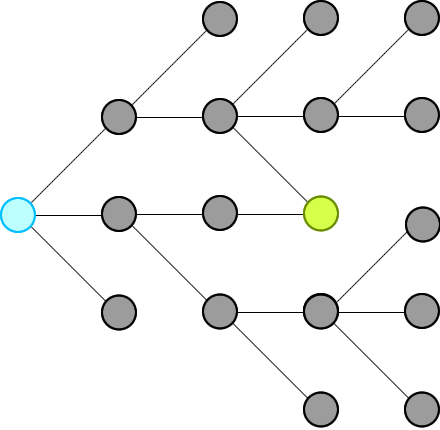We just need to record all possible words that can connect from the beginning, level by level, until we hit the end at a level.

Then we will traverse backward from end via the words in the record and construct our final ways.

Remember: **we will not record paths, we record only nodes.**


## 解释

First, because we traverse level by level, so as soon as we see the end, that is the shortest distance (shortest path) we have from beginning.

This is the basic theorem of BFS in an unweighted graph: https://sneeit.com/graph/?tab=documentation#breath-first-search-in-graph

When we see the end, we know some of the nodes from previous level (which connect to the beginning because we traversed from there) are pointing to the end.

We just need to move backward, level by level then we could collect all paths to end from begin


PS：第一次听说这个定理。

## Why Other’s Solutions Get TLE 为何其他算法超时

Because if there are some nodes point to a same node, their solutions keep computing the same path again and again due to they see those paths are different (from the beginning node). This is the weakness of recording paths, instead of nodes.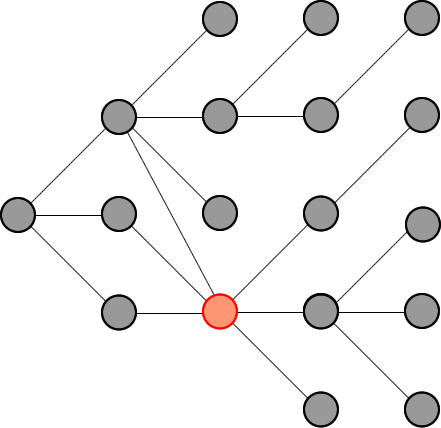In summary:

Other solutions:
Store paths, so every node could be stored multiple times.
Compute the intersections in paths again and again
Paths that does not lead to end also be computed

My solution:
Store only nodes so every node is store exactly one time
Move backward to compute only the paths that can connect from begin to end


 其他解决方案：
存储路径，因此每个节点都可以存储多次。
一次又一次地计算路径中的交叉点
不通向终点的路径也被计算

我的解决方案：
仅存储节点，因此每个节点都只存储一次
向后移动以仅计算可以从头到尾连接的路径


PS: 其实这里就是所谓的剪枝。剩下的就是要如何解决这 2 个问题了。

## 算法

Algorithm

Moving Forward: start from begin
Each level, find all connected nodes to the nodes of the current level in the record and add those to the record for the next level.
Delete node from wordList to prevent revisiting and forming cycles
Repeat the above steps until we reach end or we add no new nodes to the record for next level

Moving Backward: start from end
Do the same steps as moving forward but this time we will not record nodes but contruct our paths
Construct paths in reversing order to have paths from begin to end

 前进：从头开始

每一层，在记录中找到所有连接到当前层节点的节点，并将它们添加到下一层的记录中。

从 wordList 中删除节点以防止重新访问和形成循环

重复上述步骤直到我们到达终点或者我们没有向下一级的记录添加新节点

向后移动：从结束开始

执行与前进相同的步骤，但这次我们不会记录节点，而是构建我们的路径

以相反的顺序构建路径，使路径从头到尾


## 实现

### 伪代码

// Pseudocode
if (wordList.hasNo(endWord)) return []
wordList.delete(beginWord)

// move forward
queue = [beginWord]
paths = [] // 2D array
reached = false;
while (queue.length && !reached) {
paths.append(queue) // deep copy

// need static here to access only the nodes of this level
qlen = queue.length;
for (let i = 0; i < qlen && !reached; i++) {
from = queue.takeFirst()
forEach (to of wordList) {
if (isConnected(from, to)) {
if (to == endWord) {
reached = true
break // exit from the forEach
}

queue.push(to)
wordList.delete(to) // delete to preven a cycle
}
}
}
}

// can not reach the end eventually
if (!reached) return []

// move backward
answer = [[endWord]] // 2D array
for (level = paths.length - 1; level >= 0; level--) {
path = paths[level]
for (a = 0; a < alen; a++) {
last = p
forEach (word of path) {
if (!isConnected(last, word)) {
}

}
}
}

}

// to check if two words can connect
function isConnected(a,b) {
c = 0
for (i = 0; i < a.length && c < 2; i++) {
if (a[i] !== b[i]) c++
}
return c == 1
}


### java 实现

class Solution {
public List<List<String>> findLadders(String beginWord, String endWord, List<String> wordList) {
// boolean value indicate visited
Map<String, Boolean> wordDict = new HashMap<>();
for (String word : wordList) {
wordDict.put(word, false);
}

if (!wordDict.containsKey(endWord)) {
return new ArrayList<>();
}

// level of bfs
List<List<String>> levels = new ArrayList<>();
boolean reached = false;
wordDict.remove(beginWord);
q.offer(beginWord);

qLoop:
while (!q.isEmpty()) {
int qSize = q.size();
List<String> currentLevel = new ArrayList<>();
for (int i = 0; i < qSize; i++) {
String curr = q.poll();
if (curr.equals(endWord)) {
reached = true;
break qLoop;
}

for (String word : wordDict.keySet()) {
boolean visited = wordDict.get(word);
if (visited || !isConnected(word, curr)) continue;

wordDict.put(word, true);
q.offer(word);
}
}
}
if (!reached) {
return new ArrayList<>();
}

List<List<String>> paths = new ArrayList<>();

for (int i = levels.size() - 1; i >= 0; i--) {
List<List<String>> tmpPaths = new ArrayList<>();
List<String> currentLevel = levels.get(i);
for (List<String> path : paths) {
String curr = path.get(0);

for (String word : currentLevel) {
if (!isConnected(word, curr)) continue;
}
}
paths = tmpPaths;
}
return paths;
}

private boolean isConnected(String a, String b) {
if (a.length() != b.length()) return false;
int diffCount = 0;
for (int i = 0; i < a.length() && diffCount < 2; i++) {
if (a.charAt(i) != b.charAt(i)) {
diffCount++;
}
}
return diffCount == 1;
}
}


# 他山之石2

Share two similar Java solution that Accpted by OJ.

2.使用DFS构建从头到尾的路径。

2. 使用字符迭代来查找所有可能的路径。 不要将一个词与所有其他词进行比较，并检查它们是否仅相差一个字符。

3. 一个词只允许插入队列一次。

## java 实现

### 实现 1

public class Solution {
Map<String,List> map;

List<List> results;

public List<List> findLadders(String start, String end, Set dict) {
results= new ArrayList<List>();
if (dict.size() == 0)
return results;

int min=Integer.MAX_VALUE;

Queue<String> queue= new ArrayDeque<String>();

map = new HashMap<String,List<String>>();

for (String string:dict)

//BFS: Dijisktra search
while (!queue.isEmpty()) {

String word = queue.poll();

int step = ladder.get(word)+1;//'step' indicates how many steps are needed to travel to one word.

if (step>min) break;

for (int i = 0; i < word.length(); i++){
StringBuilder builder = new StringBuilder(word);
for (char ch='a';  ch <= 'z'; ch++){
builder.setCharAt(i,ch);
String new_word=builder.toString();

if (step>ladder.get(new_word))//Check if it is the shortest path to one word.
continue;
}else;// It is a KEY line. If one word already appeared in one ladder,
// Do not insert the same word inside the queue twice. Otherwise it gets TLE.

else{
map.put(new_word,list);
//It is possible to write three lines in one:
//Which one is better?
}

if (new_word.equals(end))
min=step;

}//End if dict contains new_word
}//End:Iteration from 'a' to 'z'
}//End:Iteration from the first to the last
}//End While

//BackTracking
backTrace(end,start,result);

return results;
}

private void backTrace(String word,String start,List<String> list){
if (word.equals(start)){
list.remove(0);
return;
}

if (map.get(word)!=null)
for (String s:map.get(word))
backTrace(s,start,list);
list.remove(0);
}

}


### 实现方式 2

Another solution using two sets.

While I found my solution more readable and efficient.

public class Solution {
List<List<String>> results;
List<String> list;
Map<String,List<String>> map;
public List<List<String>> findLadders(String start, String end, Set<String> dict) {
results= new ArrayList<List<String>>();
if (dict.size() == 0)
return results;

int curr=1,next=0;
boolean found=false;
map = new HashMap<String,List<String>>();

Queue<String> queue= new ArrayDeque<String>();
Set<String> unvisited = new HashSet<String>(dict);
Set<String> visited = new HashSet<String>();

unvisited.remove(start);
//BFS
while (!queue.isEmpty()) {

String word = queue.poll();
curr--;
for (int i = 0; i < word.length(); i++){
StringBuilder builder = new StringBuilder(word);
for (char ch='a';  ch <= 'z'; ch++){
builder.setCharAt(i,ch);
String new_word=builder.toString();
if (unvisited.contains(new_word)){
//Handle queue
if (visited.add(new_word)){//Key statement,Avoid Duplicate queue insertion
next++;
}

else{
map.put(new_word, l);
}

if (new_word.equals(end)&&!found) found=true;

}

}//End:Iteration from 'a' to 'z'
}//End:Iteration from the first to the last
if (curr==0){
if (found) break;
curr=next;
next=0;
unvisited.removeAll(visited);
visited.clear();
}
}//End While

backTrace(end,start);

return results;
}
private void backTrace(String word,String start){
if (word.equals(start)){
list.remove(0);
return;
}
if (map.get(word)!=null)
for (String s:map.get(word))
backTrace(s,start);
list.remove(0);
}
}


BFS

DFS